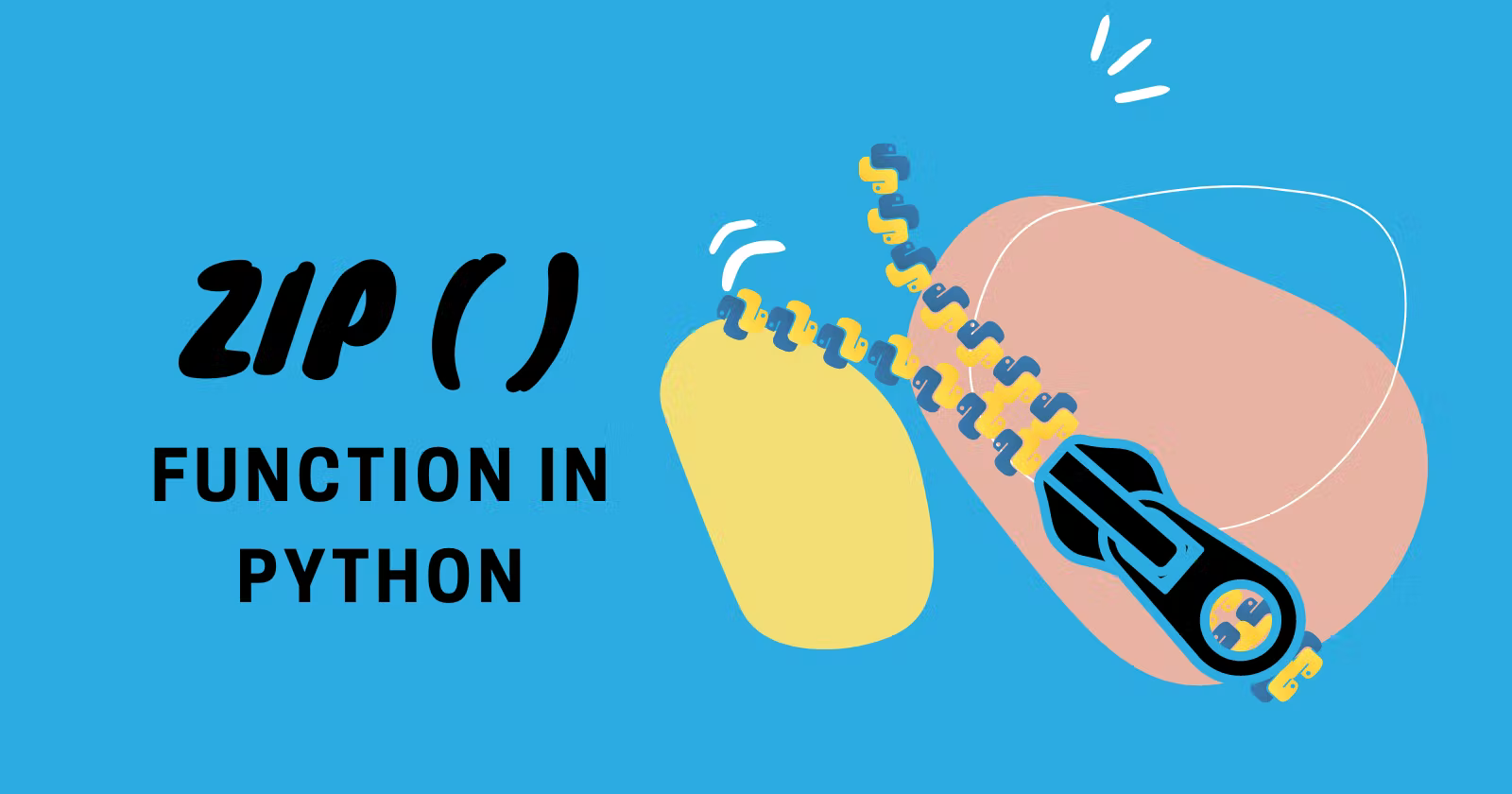## zip() Function In Python – Usage & Examples With Code

Have you ever heard the word “parallel iteration” or tried to “loop over multiple iterables in parallel” when you were coding in Python?

This tutorial will show a Python `zip()` function that helps us perform a parallel iteration over multiple iterables.

# Definition

The `zip()` function takes iterables and iterates over them parallelly, which results in producing tuples of each item from the iterables.

Output

In other words, it returns the iterator of tuples where the first item in each passed iterator is paired together and then the second item in each passed iterator is paired together and it goes so on until the shortest iterator is exhausted.

Another way to think of `zip()` is that it turns rows into columns and columns into rows. This is similar to transposing a matrix.Source

# Syntax

The syntax of Python `zip()` function is:

`zip(*iterables)` or `zip(iterator1, iterator2, ...)`

In Python 3.10, the `strict` argument was added

`zip(*iterables, strict=False)`

We’ll see the use of `strict` ahead in this tutorial.

`zip()` parameters:

`iterables`: they can be lists, tuples, dictionaries, or objects that can be iterated.

Output

# Working of zip() function

How `zip()` function creates an iterator of the tuple?

We can actually say that zipping means aggregating two separate things into one.

Just like it, the Python `zip()` function works by taking two inputs say `A1` and `A2`and then aggregating the item of the same index number of `A1` and `A2`.

We will better understand through the illustration below

• We can clearly see, on the right side – the tuple at the 0th index contains each item of `A1` and `A2` at the 0th index respectively.
• The same goes for items at the 1st index of `A1` and `A2`.
• In general, the tuple at the index `i` contains items at the index `i` in `A1` and `A2`.

More formally: zip() returns an iterator of tuples, where the i-th tuple contains the i-th element from each of the argument iterables.Source

# Iterables of different lengths

What happens if iterables are of different lengths?

Nothing’s gonna happen if the iterables passed to the `zip()` function have different lengths because if we refer to official Python docs then it states that “the iterator stops when the shortest iterable is exhausted”.

Example

Output

Here, the `C++` was excluded because the `founded` variable contains only three arguments.

### What if Python throws an error when the iterables are of different lengths?

Let’s understand with an example

Output

Did you notice that there is an additional argument `strict=True` in the code?

The `strict` argument is added in Python version 3.10

If the iterables have different lengths and we use the `strict` argument, the code will throw a `ValueError`. It can be useful for debugging.

# Passing one or no iterable to the zip()

The `zip()` function will return an empty iterator if no parameters were passed.

Output

If we pass only one iterable, then the `zip()` function will return an iterator of tuples each having only one element.

Output

# Python zip() Examples

Example: Using `enumerate()` function with `zip()`

Output

Example: Using `range()` function

Output

Example: Having multiple iterables

Output

Example: Typecasting into different data types

Typecasting into List

Output

Typecasting into Dictionary

Output

Typecasting into Set

Output

Typecasting into Tuple

Output

# Unzipping the Values

We can actually unzip the values that were already zipped. Let’s see how to do so.

This can be done with the help of `*` asterisk operator.

Let’s understand with an example.

Output

Notice how we unzipped the values of the variable `mapped` using `*` and to store the unzipped values we declared three variables `char``real` and `reel`

# Conclusion

In this tutorial, you’ve learned to perform a parallel iteration using Python’s `zip()` function and I hope you understand how to use it.

You now understand how the `zip()` function works behind the scenes to generate a tuple iterator.

Try the code snippets written above in your IDEs to understand the code better.

🏆Other articles you might be interested in if you liked this one

That’s all for now.

Keep Coding✌✌